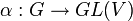# Schur functor

## Definition as a functor from vector spaces to vector spaces

### Schur functor associated with a representation of the symmetric group

Suppose$\rho:S_n \to GL(W)$ is a linear representation of the symmetric group$S_n$ over a field$K$. The Schur functor associated with$\rho$, denoted$\mathbb{S}_\rho$, is a functor from the category of$K$-vector spaces to the category of$K$-vector spaces defined as follows. First, using the equivalence of definitions of linear representation, consider$W$ as a module over the group ring$KS_n$. Now, the functor is defined as follows:

• On objects: It sends a vector space$V$ to the tensor product of modules$V^{\otimes n} \otimes_{KS_n} W$. Here is what this means.$V^{\otimes n}$ is the$n$-fold tensor product of$V$ as a$K$-vector space. This naturally acquires the structure of a$KS_n$-module where$S_n$ acts by permuting the tensor product factors. We now take its tensor product as a$KS_n$-module with$W$. This is not the same as taking the tensor product of vector spaces. Note that since$KS_n$ is not commutative, we have to treat$W$ as a left$KS_n$-module and$V^{\otimes n}$ as a right$KS_n$-module. Also note that once we have taken the tensor product, we no longer have a$KS_n$-module structure, just a$K$-vector space.
• On morphisms: Given a linear map$\mu:V_1 \to V_2$, it induces a map$\mu^{\otimes n}:V_1^{\otimes n} \to V_2^{\otimes n}$ which in turn induces a map of the tensor products with$W$. Functoriality must be checked, but is true.

### Schur functor associated with a partition

Assume that$K$ has characteristic zero. Suppose$\lambda$ is a unordered integer partition of a positive integer$n$. The Schur functor associated with$\lambda$, denoted$\mathbb{S}_\lambda$, is defined as the Schur functor associated with the irreducible linear representation of$S_n$ corresponding to$\lambda$ (see linear representation theory of symmetric groups).

In this case, the module for the irreducible representation can be thought of explicitly as a left ideal inside$KS_n$ and the tensor product operation can be thought of as multiplication on the right by this ideal. Note that multiplication by the two-sided ideal corresponding to the representation would give a sum of the Schur functor with itslf degree of the representation many times.

The result also holds if the characteristic of$K$ is greater than$n$. If$K$ has characteristic a prime less than or equal to$n$, there is some ambiguity about how to define the Schur functor.

### Combinatorial definition for finite-dimensional vector space in terms of Young tableaux

The Schur functor corresponding to a partition$\lambda$ of$n$ applied to the vector space$K^m$ (with$m$ unrelated to$n$) can be defined combinatorially as follows. As a vector space, it has basis the set of semistandard Young tableaux of shape$\lambda$ and all entries between 1 and$m$.

## Definition as a functor from representations to representations

This definition can be obtained using abstract nonsense from the definition for vector spaces. For a representation$\alpha:G \to GL(V)$ and a linear representation$\rho$ of a symmetric group$S_n$, we define$\mathbb{S}_\rho(\alpha)$ as a representation of$G$ on the vector space$S_\rho(V)$, with the map:$G \to \mathbb{S}_\rho(V)$

given by:$g \mapsto \mathbb{S}_\rho(\alpha(g))$

where the latter$\mathbb{S}_\rho$ denotes the effect of the functor on morphisms.

## Relation with Schur elements and Schur polynomials

For an unordered integer partition$\lambda$ of$n$ and an unrelated positive integer$m$, the following is the relation between the Schur functor, Schur element, and Schur polynomial:

• The Schur functor gives the vector space with basis the semistandard Young tableaux.
• The Schur element is the sum of the semistandard Young tableaux.
• The Schur polynomial is the image of the Schur element under the mapping to the polynomial ring that only remembers the multiplicity of each variable.

In particular, the dimension of the vector space obtained by applying the Schur functor can be obtained from the Schur polynomial by plugging in the value 1 for all the variables.

## Facts$V^{\otimes n} = \bigoplus_\lambda \mathbb{S}_\lambda^{d_\lambda}$

where$d_\lambda$ denotes the degree of the irreducible representation of$S_n$ corresponding to$\lambda$. The notation here means that$\mathbb{S}_\lambda$ is added to itself$d_\lambda$ times.

Note that although$\mathbb{S}_\lambda$ does not have a natural$S_n$-action,$\mathbb{S}_\lambda^{d_\lambda}$ does.